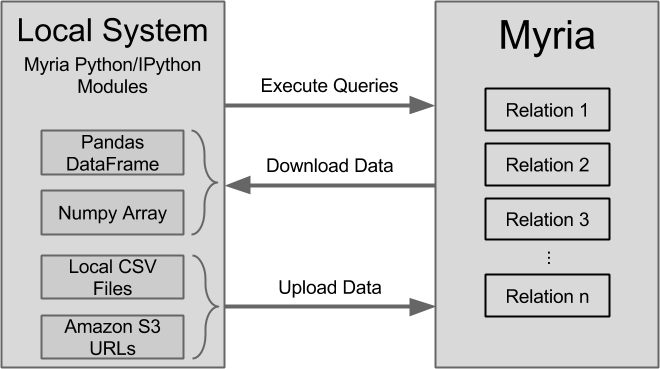# Myria Python

Myria-Python is a Python interface to the Myria project, a distributed, shared-nothing big data management system and Cloud service from the University of Washington.

The Python components include intuitive, high-level interfaces for working with Myria, along with lower-level operations for interacting directly with the Myria API.

Developers interact with the Myria system using `MyriaConnection` instances to establish a connection to the database, `MyriaQuery` instances to issue queries and obtain results, and `MyriaRelation` instances to interact with stored data. Data may be uploaded in a variety of formats via a URL or the local file system. Downloaded data may be easily converted into Python dictionaries, Pandas dataframes, and Numpy arrays. A general workflow might involve the following high-level steps:Myria-Python is also compatible with Jupyter (IPython) Notebooks. See the section below for examples.

#### Quick Start Example

The following example illustrates a subset of the functionality available in the Myria Python library:

``````from myria import *

## Establish a default connection to Myria
MyriaRelation.DefaultConnection = MyriaConnection(rest_url='http://demo.myria.cs.washington.edu:8753')

## Higher-level interaction via relation and query instances
query = MyriaQuery.submit(
csv(schema(name:string, pages:int)));
longerBooks = [from books where pages > 300 emit name];
store(longerBooks, LongerBooks);""")

json = query.to_dict()

dataframe = query.to_dataframe()

dataframe = query.to_dataframe().as_matrix()

relation = MyriaRelation(relation='LongerBooks')
print len(relation)

## Lower-level interaction via the REST API
connection = MyriaConnection(rest_url='http://demo.myria.cs.washington.edu:8753')
datasets = connection.datasets()
``````

## Installation

Users can install the Python libraries using `pip install myria-python`. Developers should clone the repository and run `python setup.py develop`.

## Using Python with the Myria Service

### Part 1: Running Queries

In this Python example, we query the smallTable relation by creating a `count(*)` query. In this query, we store our result to a relation called dataCount. To learn more about the Myria query language, check out the MyriaL page.

``````from myria import *
MyriaRelation.DefaultConnection = MyriaConnection(rest_url='http://demo.myria.cs.washington.edu:8753')

query = MyriaQuery.submit("""
csv(schema(left:int, right:int)));
q = [from data emit count(*)];
store(q, dataCount);""")

print query.to_dict()
``````

In the previous example we downloaded the result of a query. We can also download data that has been stored as a relation:

``````from myria import *
MyriaRelation.DefaultConnection = MyriaConnection(rest_url='http://demo.myria.cs.washington.edu:8753')

# Load some data and store it in Myria
query = MyriaQuery.submit("""
csv(schema(left:int, right:int)));
store(data, data);""")

# Now access previously-stored data
relation = MyriaRelation('data')

print relation.to_dict()[:5]
``````

#### 1. From a local Python variable

``````from myria import *

schema = { "columnNames" : ["name", "pages"],
"columnTypes" : ["STRING_TYPE","LONG_TYPE"] }

data = """Brave New World,288
Nineteen Eighty-Four,376
We,256"""

connection = MyriaConnection(rest_url='http://demo.myria.cs.washington.edu:8753')
name, schema, data, delimiter=',', overwrite=True)

relation = MyriaRelation("Books", connection=connection)
print relation.to_dict()
``````

#### 2. From a Local File

``````import sys
import urllib
import random
from myria import *

connection = MyriaConnection(rest_url='http://demo.myria.cs.washington.edu:8753')

urllib.urlretrieve ("https://raw.githubusercontent.com/uwescience/myria-python/master/ipnb%20examples/books.csv",
"books.csv")

# Initialize a name and schema for the new relation
'relationName': 'Books' + str(random.randrange(sys.maxint)) } # Name must be unique!
schema = { "columnNames" : ["name", "pages"],
"columnTypes" : ["STRING_TYPE","LONG_TYPE"] }

# Now upload that file to Myria
with open('books.csv') as f:

# Now access the new relation
relation = MyriaRelation(name, connection=connection)
print relation.to_dict()
``````

#### 3. From the Command Line

In the example below, we upload a local CSV file to the Myria Service. Here is an example you can run through your terminal (assuming you’ve setup myria-python):

``````wget https://raw.githubusercontent.com/uwescience/myria/master/jsonQueries/getting_started/smallTable
myria_upload --overwrite --hostname demo.myria.cs.washington.edu --port 8753 --no-ssl --relation smallTable smallTable
``````

Myria can upload a relation in parallel. Each worker must point to a partition of the file. Users must either create or have these partitions prepared in S3. In the example below, worker 1 reads the first part of the file (TwitterK-part1.csv) while worker 2 reads the last part of the file (TwitterK-part2.csv).

``````from myria import *
MyriaRelation.DefaultConnection = MyriaConnection(rest_url='http://demo.myria.cs.washington.edu:8753')

schema = MyriaSchema({"columnTypes" : ["LONG_TYPE", "LONG_TYPE"], "columnNames" : ["follower", "followee"]})

# A list of worker-URL pairs -- must be one for each worker

query = MyriaQuery.parallel_import(relation=relation, work=work)

print query.status
``````

### Part 4. Python Expressions

Myria allows arbitrary Python Functions as expressions which can be used in transformations like map, flatmap and aggregates.

#### Registering a Python Function

Python function needs to be registered before it can be used. Myria-Python can be used to register new Python functions, list existing registered Python functions, and to retrieve details about existing registered functions.

``````from myria.udf import MyriaFunction, MyriaPythonFunction
from raco.types import LONG_TYPE

#create connection
MyriaRelation.DefaultConnection = MyriaConnection(rest_url='http://demo.myria.cs.washington.edu:8753')

#register Python functions
@myria_function(output_type="LONG_TYPE")
def pyIsPrime(dt):
import math
n = dt
if n % 2 == 0 and n > 2:
return False
for i in range(3, int(math.sqrt(n)) + 1, 2):
if n % i == 0:
return 0
return 1

#List registered functions
print MyriaFunction.get_all()

#List details of a registered function
print MyriaFunction.get('pyIsPrime')

``````

#### Invoking Python Expression in MyriaL

A registered Python function can then be used in a MyriaL query anywhere an expression can be used.

``````q = MyriaQuery.submit("""
isPrime = [from T1 emit pyIsPrime(T1.src) as isPrime, T1.src, T1.dst];
""")
q.status

``````

#### Python Expressions in UDAs

Python functions can also be used in User Defined Aggregates in MyriaL.

``````#register a python function
@myria_function(output_type="LONG_TYPE")
def udfSum(dt):
tuplist = dt
state = 0
for i in tuplist:
state +=1
return state

#define a UDA
q = MyriaQuery.submit("""
uda aggregate(a) {
--init
[ 0 as total];
--update
[udfSum(a)];
--emit
[total];
};
results = [from t emit t.a,  aggregate(t.b) as total];
store(results, results);
""", connection=connection)

q.status
``````

## Using Myria with IPython

Myria exposes convenience functionality when running within the Jupyter/IPython environment. See our sample IPython notebook for a live demo.

``````%load_ext myria
``````

#### 2. Connect to Myria

``````%connect http://demo.myria.cs.washington.edu:8753
``````

#### 3. Execute Queries

``````%%query
csv(schema(name:string, pages:int)));
longerBooks = [from books where pages > 300 emit name];
store(longerBooks, LongerBooks);
``````

#### 4. Variable Binding

You can embed local Python variables into a query expression. For example, assume we have set the following local variables:

``````low, high, name = 300, 1000, 'MyBooks'
``````

Now we can execute a query in an IPython notebook that binds over our local environment:

``````%%query